6.2.5

# Dynamic SNAT

Dynamic SNAT maps the private IP addresses to the first available public address from a pool of addresses. In the FortiGate firewall, this can be done by using IP pools. IP pools is a mechanism that allows sessions leaving the FortiGate firewall to use NAT. An IP pool defines a single IP address or a range of IP addresses to be used as the source address for the duration of the session. These assigned addresses are used instead of the IP address assigned to that FortiGate interface.

## IP pool types

FortiGate uses four types of IPv4 IP pools. This recipe focuses on some of the differences between them.

This type of IP pool is similar to static SNAT mode. We need to define an external IP range that contains one or more IP addresses. When there is only one IP address it is almost the same as static SNAT, the outgoing interface address is used. When it contains multiple IP addresses, it is equivalent to an extended mode of static SNAT.

For instance, if we define an overload type IP pool with two external IP addresses (172.16.200.1—172.16.200.2), since there are 60,416 available port numbers per IP, this IP pool can handle 60,416*2 internal IP addresses.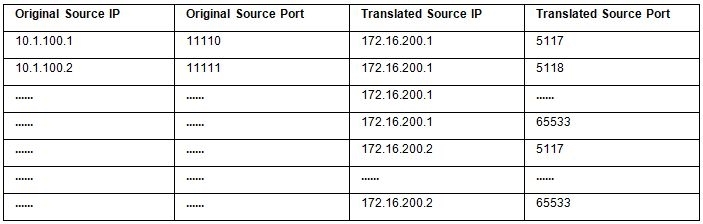The mapped IP address can be calculated from the source IP address. The index number of the address in the pool is the remainder of the source IP address, in decimal, divided by the number addresses in the pool.To calculate the decimal value of the source IP address, either use an online calculator, or use the following equation: a.b.c.d = a * (256)3 + b * (256)2 + c * (256) + d For example: 192.168.0.1 = 192 * (256)3 + 168 * (256)2 + 0 * (256) + 1 = 3232235521

If there is one IP pool, where:

• P1 = the first address in the IP pool
• R1 = the number of IP addresses in the IP pool
• X = the source IP address as a decimal number
• Y = the mapped IP address

Then the equation to determine the mapped address is:

Y = P1 + X mod R1

For example:

IP pool

172.26.73.20 to 172.26.73.90

192.168.1.200

1. Convert the source IP address to a decimal number:

192 * (256)3 + 168 * (256)2 + 1 * (256) + 200 = 3232235976

2. Determine the number of IP addresses in the pool:

172.26.73.90 - 172.26.73.20 = 71

3. Find the remainder of the source IP address divided by the number of addresses in the pool:

3232235976 mod 71 = 26

4. Add the remainder to the first IP address in the pool:

172.26.73.20 + 26 = 172.26.73.46

So, the mapped IP address is 172.26.73.46.

If there are multiple IP pools, the calculation is similar to when there is only one pool.

If there are two IP pools, where:

• P1 = the first address in the first IP pool
• P2 = the first address in the second IP pool
• R1 = the number of IP addresses in the first IP pool
• R2 = the number of IP addresses in the second IP pool
• X = the source IP address as a decimal number
• Y = the mapped IP address

Then the equations to determine the mapped address are:

If X mod (R1 + R2) >= R1, then Y = P2 + X mod R2

If X mod (R1 + R2) < R1, then Y = P1 + X mod R1

For example:

IP pools

pool01: 172.26.73.20 to 172.26.73.90

192.168.1.200

pool02: 172.26.75.50 to 172.26.75.150

1. Convert the source IP address to a decimal number:

192 * (256)3 + 168 * (256)2 + 1 * (256) + 200 = 3232235976

2. Determine the total number of IP addresses in the pools:

(172.26.73.90 - 172.26.73.20) + (172.26.75.50 - 172.26.75.150) = 71 + 101 = 172

3. Find the remainder of the source IP address divided by the number of addresses in the pools:

3232235976 mod 172 = 108

4. The remainder is greater than the number of addresses in pool01, so the address is selected from pool02 and the remainder is recalculated based only on pool02:

3232235976 mod 101 = 40

5. Add the new remainder to the first IP address in pool02:

172.26.75.50 + 40 = 172.26.75.90

So, the mapped IP address is 172.26.75.90.

### One-to-one

This type of IP pool means that the internal IP address and the external (translated) IP address match one-to-one. The port address translation (PAT) is disabled when using this type of IP pool. For example, if we define a one-to-one type IP pool with two external IP addresses (172.16.200.1 - 172.16.200.2), this IP pool only can handle two internal IP addresses.

### Fixed port range

For the overload and one-to-one IP pool types, we do not need to define the internal IP range. For the fixed port range type of IP pool, we can define both internal IP range and external IP range. Since each external IP address and the number of available port numbers is a specific number, if the number of internal IP addresses is also determined, we can calculate the port range for each address translation combination. So we call this type fixed port range. This type of IP pool is a type of port address translation (PAT).

For instance, if we define one external IP address (172.16.200.1) and ten internal IP addresses (10.1.100.1-10.1.100.10), we have translation IP+Port combination like following table: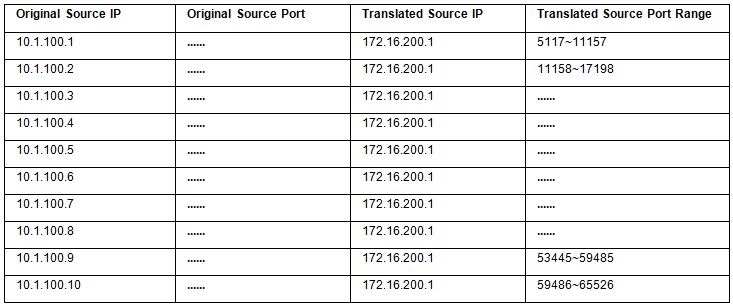### Port block allocation

This type of IP pool is also a type of port address translation (PAT). It gives users a more flexible way to control the way external IPs and ports are allocated. Users need to define Block Size/Block Per User and external IP range. Block Size means how many ports each Block contains. Block per User means how many blocks each user (internal IP) can use.

The following is a simple example:

• External IP Range: 172.16.200.1—172.16.200.1
• Block Size: 128
• Block Per User: 8

Result:

• Total-PBAs: 472 (60416/128)
• Maximum ports can be used per User (Internal IP Address): 1024 (128*8)
• How many Internal IP can be handled: 59 (60416/1024 or 472/8)

## Sample configuration

###### To configure overload IP pool in the GUI:
1. In Policy & Objects > IP Pools, click Create New.
2. Select IPv4 Pool and then select Overload.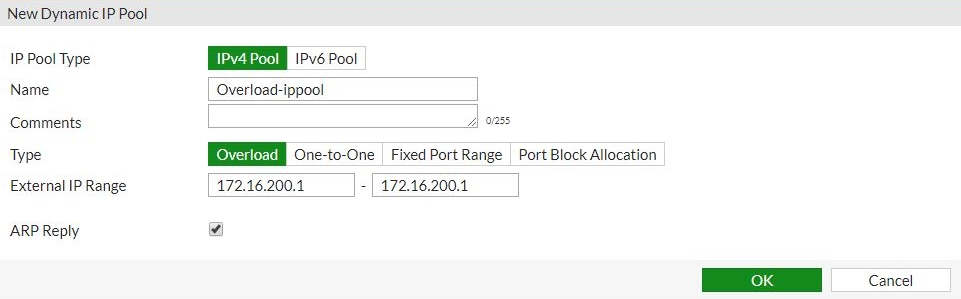###### To configure overload IP pool in the CLI:
```config firewall ippool
set startip 172.16.200.1
set endip 172.16.200.1
next
end```
###### To configure one-to-one IP pool using the GUI:
1. In Policy & Objects > IP Pools, click Create New.
2. Select IPv4 Pool and then select One-to-One.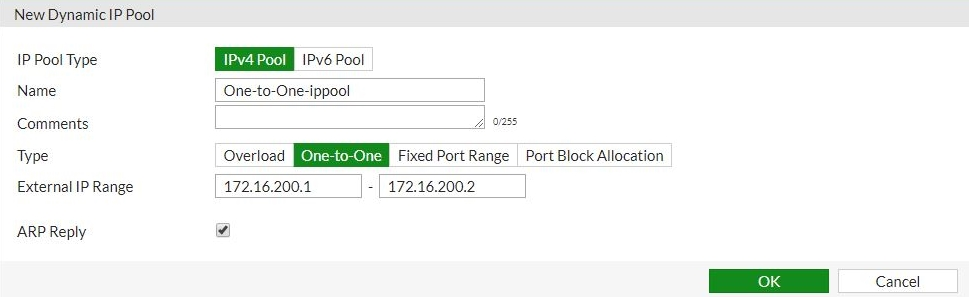###### To configure one-to-one IP pool in the CLI:
```config firewall ippool
edit "One-to-One-ippool"
set type one-to-one
set startip 172.16.200.1
set endip 172.16.200.2
next
end```
###### To configure fixed port range IP pool in the GUI:
1. In Policy & Objects > IP Pools, click Create New.
2. Select IPv4 Pool and then select Fixed Port Range.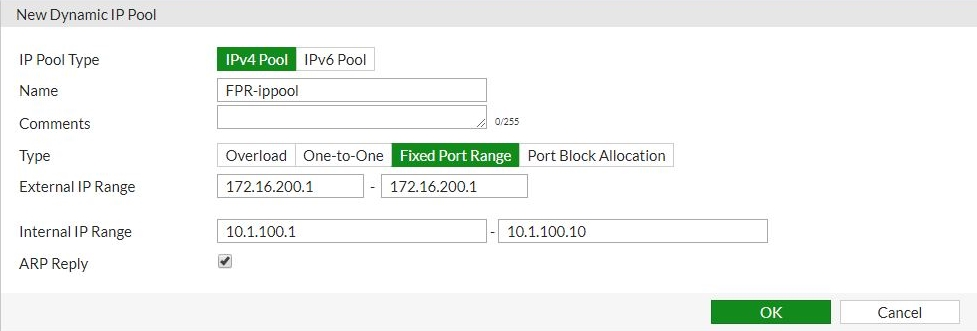###### To configure fixed port range IP pool in the CLI:
```config firewall ippool
edit "FPR-ippool"
set type fixed-port-range
set startip 172.16.200.1
set endip 172.16.200.1
set source-startip 10.1.100.1
set source-endip 10.1.100.10
next
end```
###### To configure port block allocation IP pool in the GUI:
1. In Policy & Objects > IP Pools, click Create New.
2. Select IPv4 Pool and then select Port Block Allocation.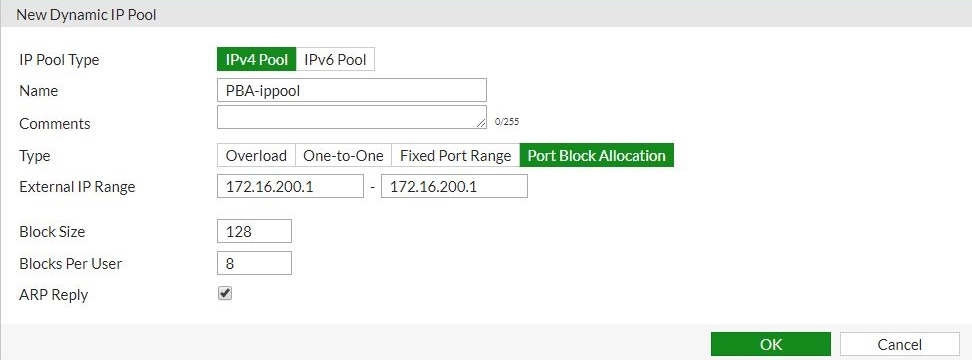###### To configure port block allocation IP pool in the CLI:
```config firewall ippool
edit PBA-ippool
set type port-block-allocation
set startip 172.16.200.1
set endip 172.16.200.1
set block-size 128
set num-blocks-per-user 8
next
end```

# Dynamic SNAT

Dynamic SNAT maps the private IP addresses to the first available public address from a pool of addresses. In the FortiGate firewall, this can be done by using IP pools. IP pools is a mechanism that allows sessions leaving the FortiGate firewall to use NAT. An IP pool defines a single IP address or a range of IP addresses to be used as the source address for the duration of the session. These assigned addresses are used instead of the IP address assigned to that FortiGate interface.

## IP pool types

FortiGate uses four types of IPv4 IP pools. This recipe focuses on some of the differences between them.

This type of IP pool is similar to static SNAT mode. We need to define an external IP range that contains one or more IP addresses. When there is only one IP address it is almost the same as static SNAT, the outgoing interface address is used. When it contains multiple IP addresses, it is equivalent to an extended mode of static SNAT.

For instance, if we define an overload type IP pool with two external IP addresses (172.16.200.1—172.16.200.2), since there are 60,416 available port numbers per IP, this IP pool can handle 60,416*2 internal IP addresses.The mapped IP address can be calculated from the source IP address. The index number of the address in the pool is the remainder of the source IP address, in decimal, divided by the number addresses in the pool.To calculate the decimal value of the source IP address, either use an online calculator, or use the following equation: a.b.c.d = a * (256)3 + b * (256)2 + c * (256) + d For example: 192.168.0.1 = 192 * (256)3 + 168 * (256)2 + 0 * (256) + 1 = 3232235521

If there is one IP pool, where:

• P1 = the first address in the IP pool
• R1 = the number of IP addresses in the IP pool
• X = the source IP address as a decimal number
• Y = the mapped IP address

Then the equation to determine the mapped address is:

Y = P1 + X mod R1

For example:

IP pool

172.26.73.20 to 172.26.73.90

192.168.1.200

1. Convert the source IP address to a decimal number:

192 * (256)3 + 168 * (256)2 + 1 * (256) + 200 = 3232235976

2. Determine the number of IP addresses in the pool:

172.26.73.90 - 172.26.73.20 = 71

3. Find the remainder of the source IP address divided by the number of addresses in the pool:

3232235976 mod 71 = 26

4. Add the remainder to the first IP address in the pool:

172.26.73.20 + 26 = 172.26.73.46

So, the mapped IP address is 172.26.73.46.

If there are multiple IP pools, the calculation is similar to when there is only one pool.

If there are two IP pools, where:

• P1 = the first address in the first IP pool
• P2 = the first address in the second IP pool
• R1 = the number of IP addresses in the first IP pool
• R2 = the number of IP addresses in the second IP pool
• X = the source IP address as a decimal number
• Y = the mapped IP address

Then the equations to determine the mapped address are:

If X mod (R1 + R2) >= R1, then Y = P2 + X mod R2

If X mod (R1 + R2) < R1, then Y = P1 + X mod R1

For example:

IP pools

pool01: 172.26.73.20 to 172.26.73.90

192.168.1.200

pool02: 172.26.75.50 to 172.26.75.150

1. Convert the source IP address to a decimal number:

192 * (256)3 + 168 * (256)2 + 1 * (256) + 200 = 3232235976

2. Determine the total number of IP addresses in the pools:

(172.26.73.90 - 172.26.73.20) + (172.26.75.50 - 172.26.75.150) = 71 + 101 = 172

3. Find the remainder of the source IP address divided by the number of addresses in the pools:

3232235976 mod 172 = 108

4. The remainder is greater than the number of addresses in pool01, so the address is selected from pool02 and the remainder is recalculated based only on pool02:

3232235976 mod 101 = 40

5. Add the new remainder to the first IP address in pool02:

172.26.75.50 + 40 = 172.26.75.90

So, the mapped IP address is 172.26.75.90.

### One-to-one

This type of IP pool means that the internal IP address and the external (translated) IP address match one-to-one. The port address translation (PAT) is disabled when using this type of IP pool. For example, if we define a one-to-one type IP pool with two external IP addresses (172.16.200.1 - 172.16.200.2), this IP pool only can handle two internal IP addresses.

### Fixed port range

For the overload and one-to-one IP pool types, we do not need to define the internal IP range. For the fixed port range type of IP pool, we can define both internal IP range and external IP range. Since each external IP address and the number of available port numbers is a specific number, if the number of internal IP addresses is also determined, we can calculate the port range for each address translation combination. So we call this type fixed port range. This type of IP pool is a type of port address translation (PAT).

For instance, if we define one external IP address (172.16.200.1) and ten internal IP addresses (10.1.100.1-10.1.100.10), we have translation IP+Port combination like following table:### Port block allocation

This type of IP pool is also a type of port address translation (PAT). It gives users a more flexible way to control the way external IPs and ports are allocated. Users need to define Block Size/Block Per User and external IP range. Block Size means how many ports each Block contains. Block per User means how many blocks each user (internal IP) can use.

The following is a simple example:

• External IP Range: 172.16.200.1—172.16.200.1
• Block Size: 128
• Block Per User: 8

Result:

• Total-PBAs: 472 (60416/128)
• Maximum ports can be used per User (Internal IP Address): 1024 (128*8)
• How many Internal IP can be handled: 59 (60416/1024 or 472/8)

## Sample configuration

###### To configure overload IP pool in the GUI:
1. In Policy & Objects > IP Pools, click Create New.
2. Select IPv4 Pool and then select Overload.###### To configure overload IP pool in the CLI:
```config firewall ippool
set startip 172.16.200.1
set endip 172.16.200.1
next
end```
###### To configure one-to-one IP pool using the GUI:
1. In Policy & Objects > IP Pools, click Create New.
2. Select IPv4 Pool and then select One-to-One.###### To configure one-to-one IP pool in the CLI:
```config firewall ippool
edit "One-to-One-ippool"
set type one-to-one
set startip 172.16.200.1
set endip 172.16.200.2
next
end```
###### To configure fixed port range IP pool in the GUI:
1. In Policy & Objects > IP Pools, click Create New.
2. Select IPv4 Pool and then select Fixed Port Range.###### To configure fixed port range IP pool in the CLI:
```config firewall ippool
edit "FPR-ippool"
set type fixed-port-range
set startip 172.16.200.1
set endip 172.16.200.1
set source-startip 10.1.100.1
set source-endip 10.1.100.10
next
end```
###### To configure port block allocation IP pool in the GUI:
1. In Policy & Objects > IP Pools, click Create New.
2. Select IPv4 Pool and then select Port Block Allocation.###### To configure port block allocation IP pool in the CLI:
```config firewall ippool
edit PBA-ippool
set type port-block-allocation
set startip 172.16.200.1
set endip 172.16.200.1
set block-size 128
set num-blocks-per-user 8
next
end```